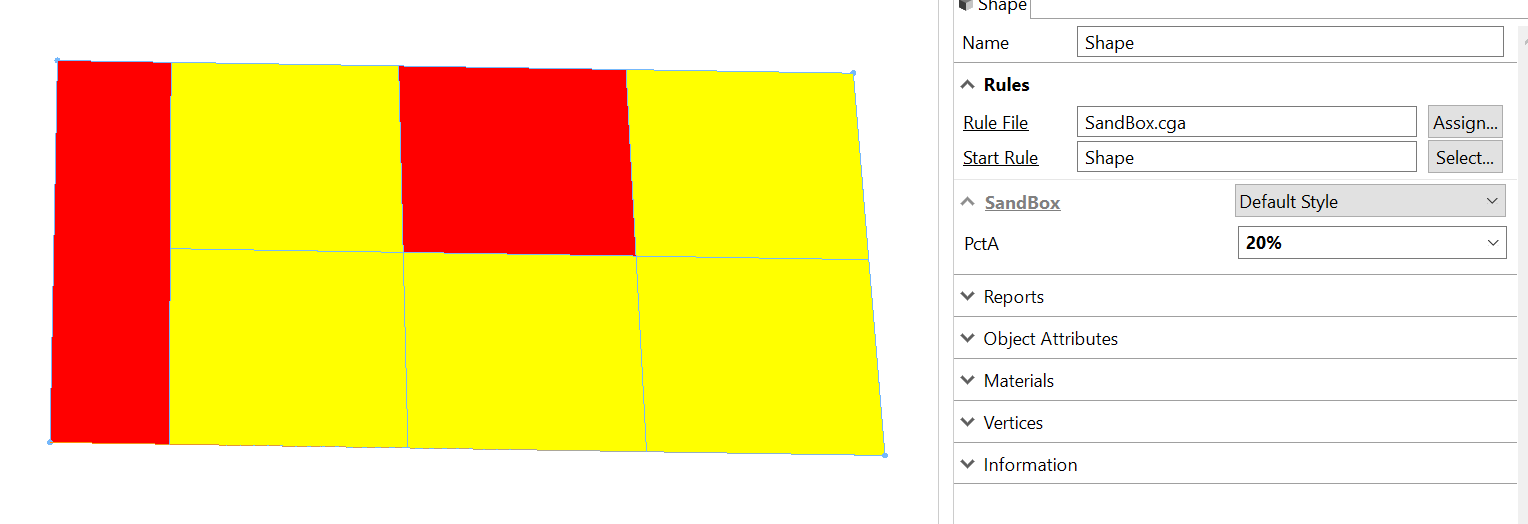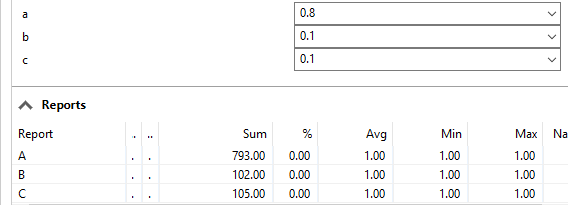# How can I use attributes as precentages in a stochastic rule

1104
4
04-22-2019 08:58 PMNew Contributor III

How can I do this:

` @Range(min=0, max=1, stepsize=0.1, restricted=true) @Percent`

`attr percentage_of_A = 0.5`

`Generate -->`

`   percentage_of_A : A`

`   else: B `

Thank you

Theo

Tags (2)
4 RepliesOccasional Contributor III

Wow you are really close. This solution is not well document. I used to have to refer to some other rules every time I was trying to figure this out. What you are looking for is the p function (probability function).  Essentially you are testing a probability and will use it with a conditional rule.

``````@Range(min=0, max=1, stepsize=0.1, restricted=true) @Percent

attr PctA = 0.5

Generate -->
case p(PctA): color(1,0,0) A
else:         color(1,1,0) B‍‍‍‍‍‍‍``````by Anonymous User
Not applicable

Thanks Devin!

To take this topic further, Cheryl Lau‌ has a good explanation how the p() function used multiple times will not work as it might seem to, and her code example shows an excellent way of getting around that. Here's Cheryl's example:New Contributor III

Thanks Devin Lavigne‌ and Chris Wilkins

I tested Cheryl Lau‌ 's workaround with 3 different set of values:

 Rule to Apply User Percentage Generated Percentage A 0.25 0.404 B 0.40 0.325 C 0.35 0.271 A 0.80 0.815 B 0.10 0.102 C 0.10 0.083 A 0.30 0.373 B 0.35 0.323 C 0.35 0.304

In most cases it's pretty close, but it sometime deviates significantly.

In my case the percentages are defined by the user via the inspector so I might have to consider hard coding the values in the rule and asking the user to edit the rule file directly.by Anonymous User
Not applicable

Theodore,

How many times was the rule run to get those numbers?  I ask because both Cheryl Lau‌'s rule and the Stochastic rule (with it's hard-coded percentages) work using randomness, and they are not dictating exact percentages. From CGA reference:

"All random numbers, also the choice of the percentages ..., depend on the current shape's seed (the seedian shape attribute)."

The stochastic functions will not guarantee those percentages, but if you run it very many times, they will begin to average out to those numbers. Also, unless you hit "Update Seed", you'll always get same results.

I ran both hard and soft-coded versions 1000 times and average was very close.

Chris

Here's Cheryl's code with a loop added:

``````attr a = 0.8
attr b = 0.1
attr c = 0.1

howMany = 1000

@StartRule
Rule1 -->
LoopOnRule2(0)

LoopOnRule2(loopCount) -->
case loopCount < howMany:
Rule2(rand(0,1))
LoopOnRule2(loopCount+1)
else: NIL

Rule2(x) -->
case x<a:                     report("A",1)
case x>=a && x<a+b:           report("B",1)
case x>=a+b && x<=a+b+c:		report("C",1)
else:							report("ELSE",1) # This won't happen. ‍‍‍‍‍‍‍‍‍‍‍‍‍‍‍‍‍‍‍‍‍``````

Does this explain it any further? Is the looping rule clear?

Here's one run of the above code, which shows results in the Reports panel of the Inspector:Hope this helps,

Chris